# Selina Solutions Concise Maths Class 10 Chapter 1 GST [Goods and Services Tax]

Taxes are computed on the cost of purchase of goods or services or both, at a percentage pronounced by the government. So in this Chapter, students will get a brief idea about the computation of such taxes. Students who find it difficult to solve the problems of this Chapter can use the Selina Solutions for Class 10 Maths. We at BYJU’S, having a wide experience in the education industry have created these Selina Solutions to meet the requirements of students.
This Chapter contains two exercises. The problems pertain to GST tax calculation and Input Tax Credit (ITC). Students can make use of Selina Solutions Concise Maths Class 10 Chapter 1 GST [Goods and Services Tax] PDF from the link provided below.

## Selina Solutions Concise Maths Class 10 Chapter 1 GST [Goods and Services Tax] Download PDF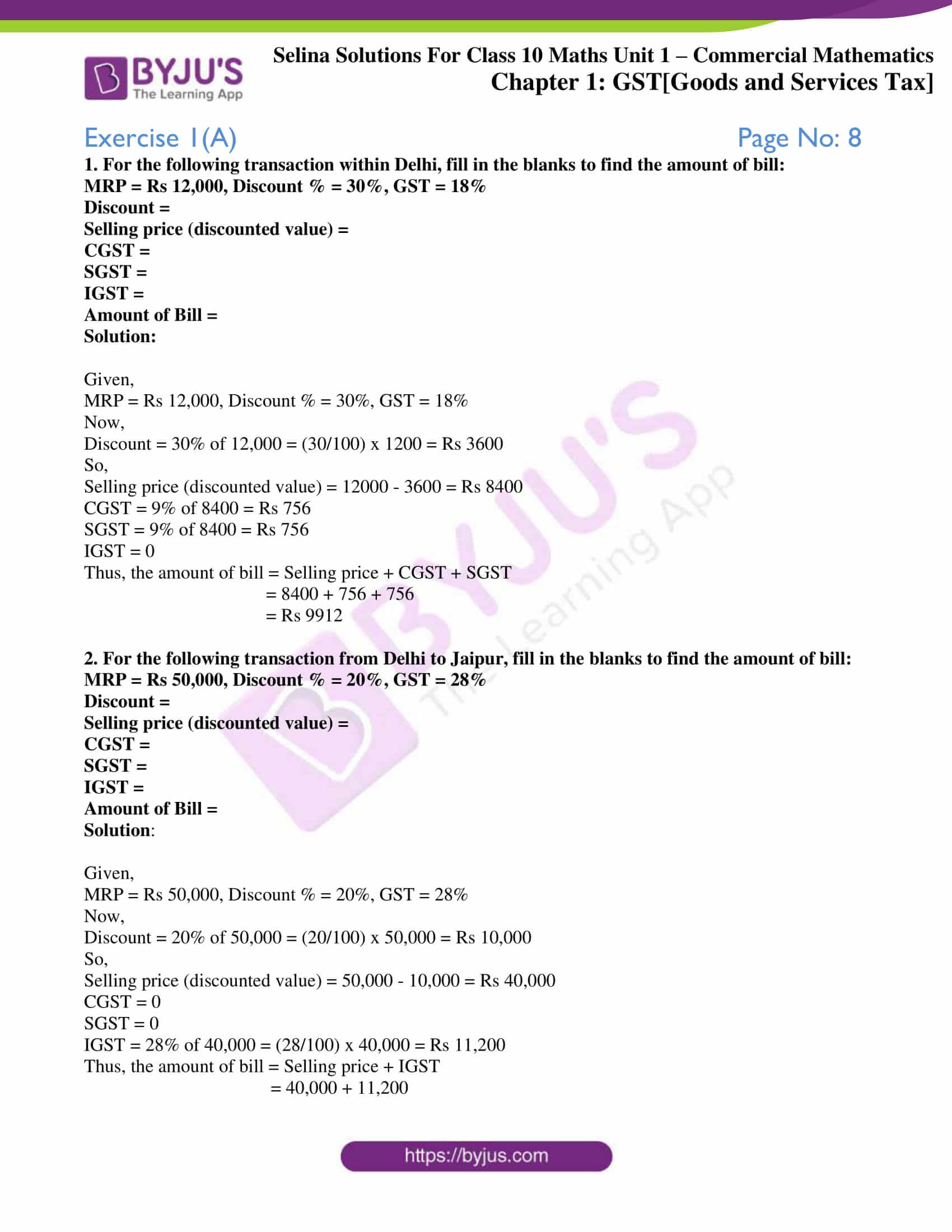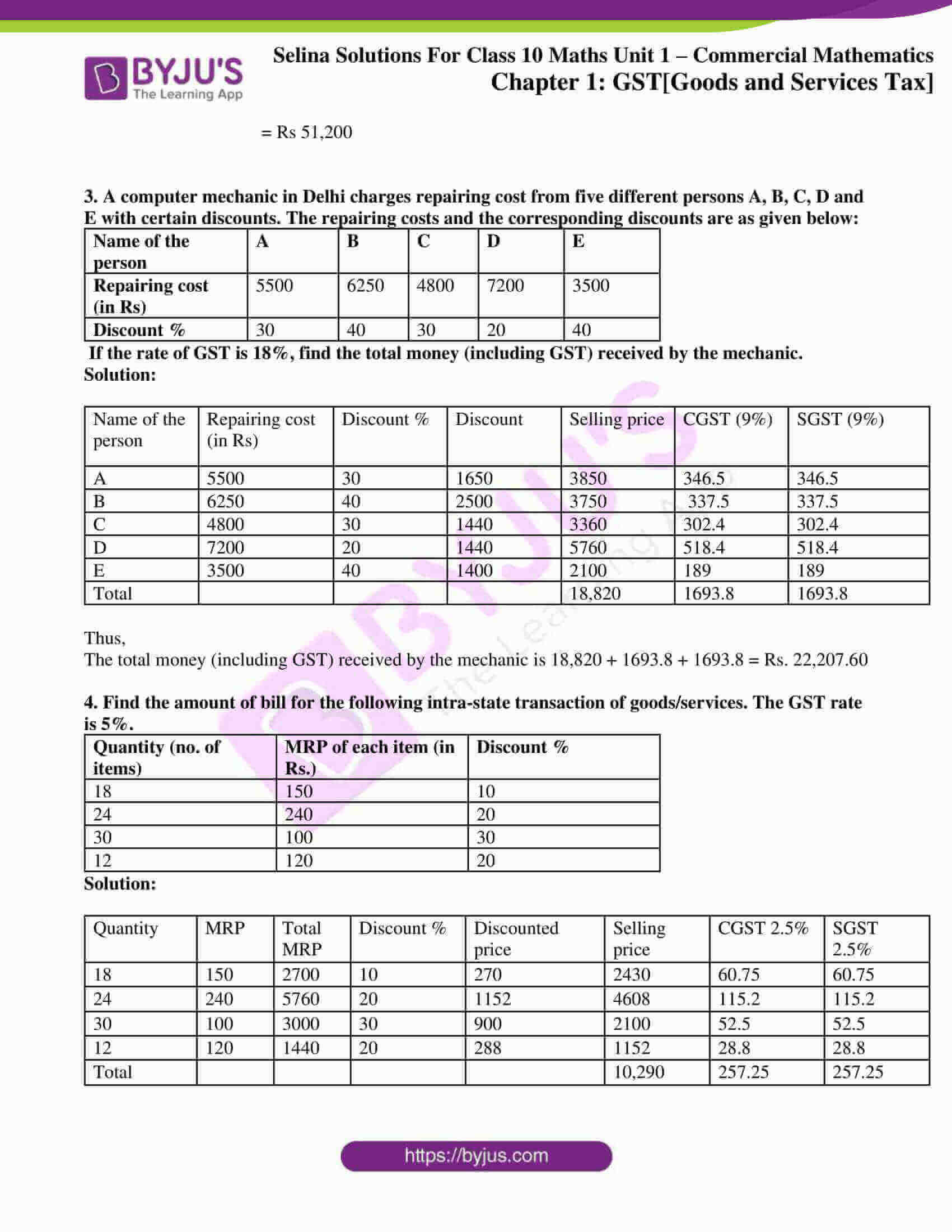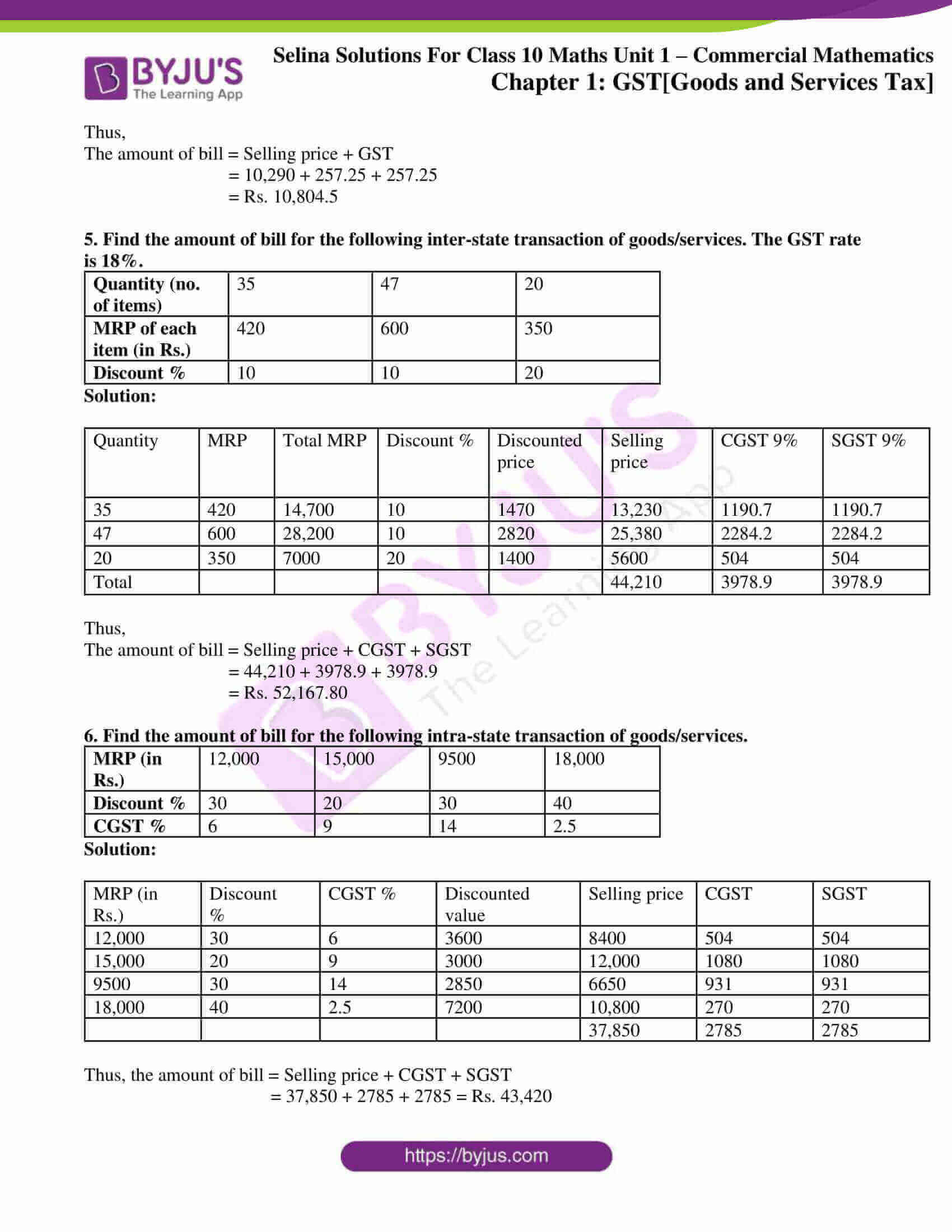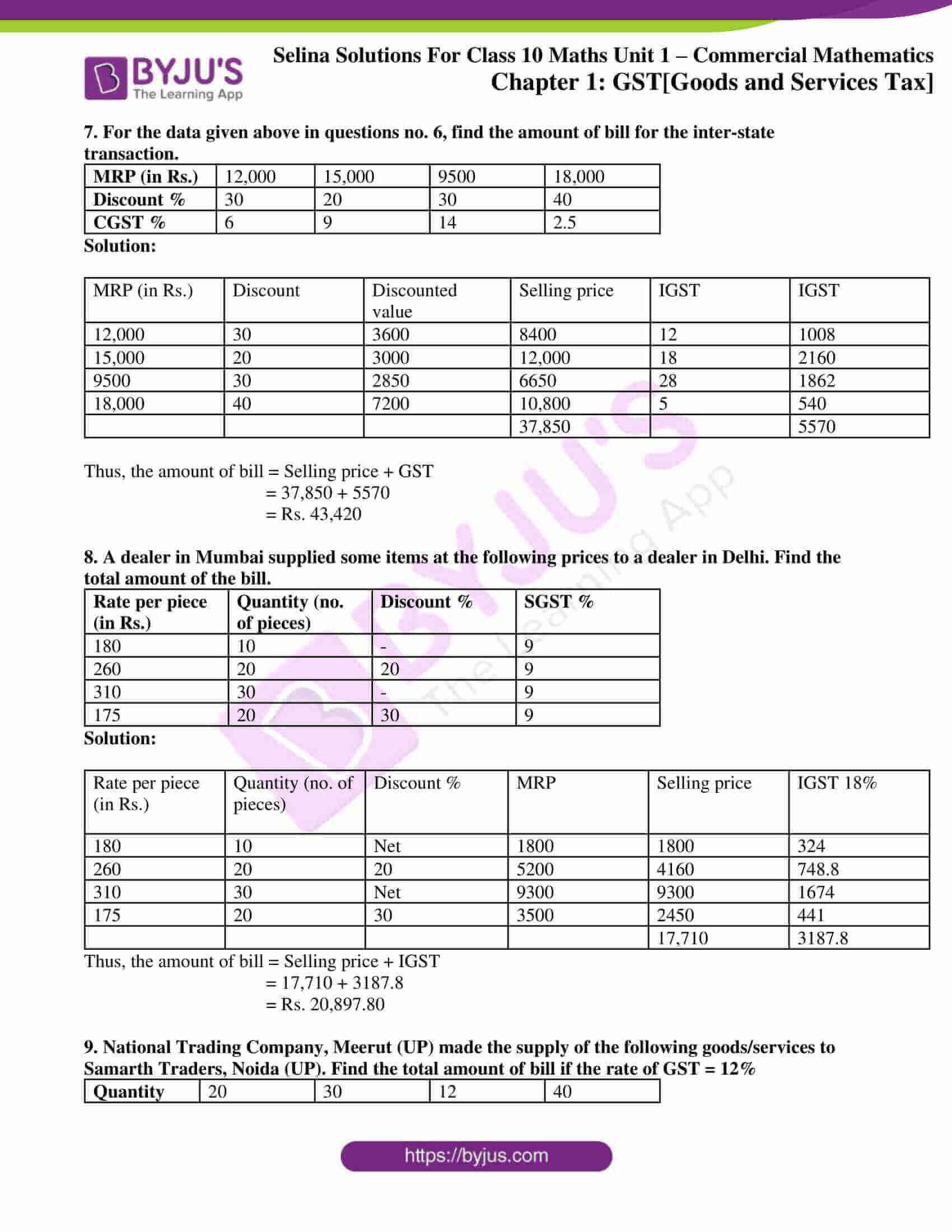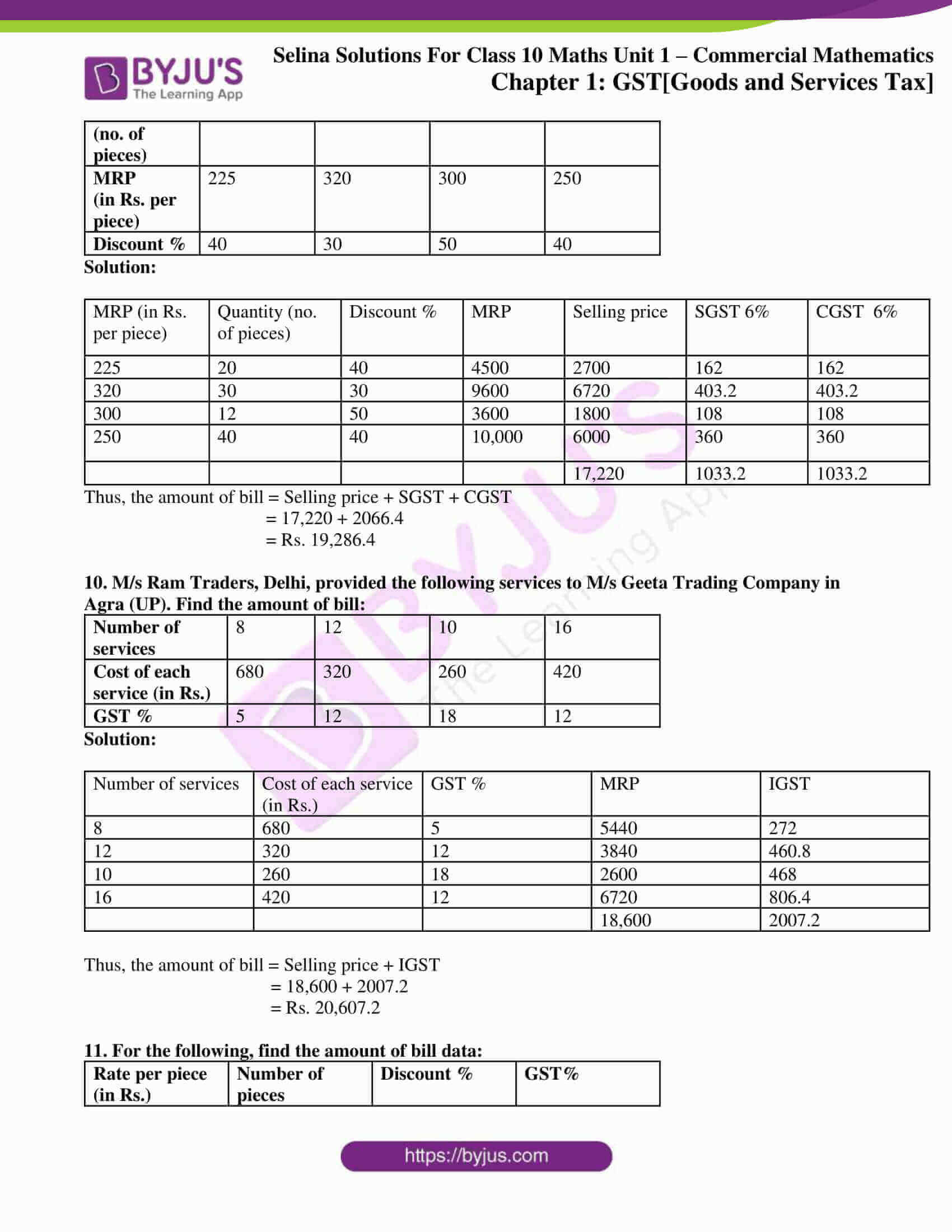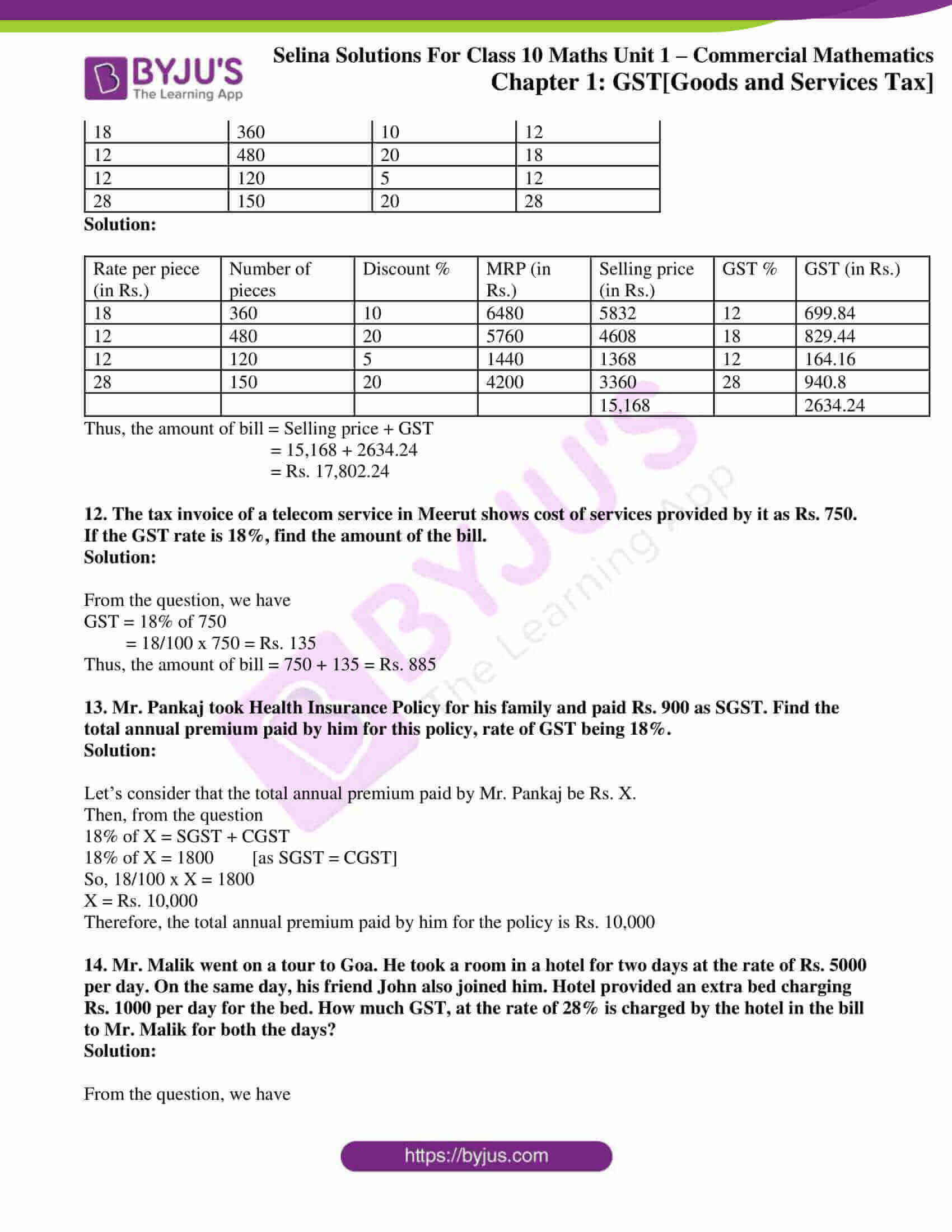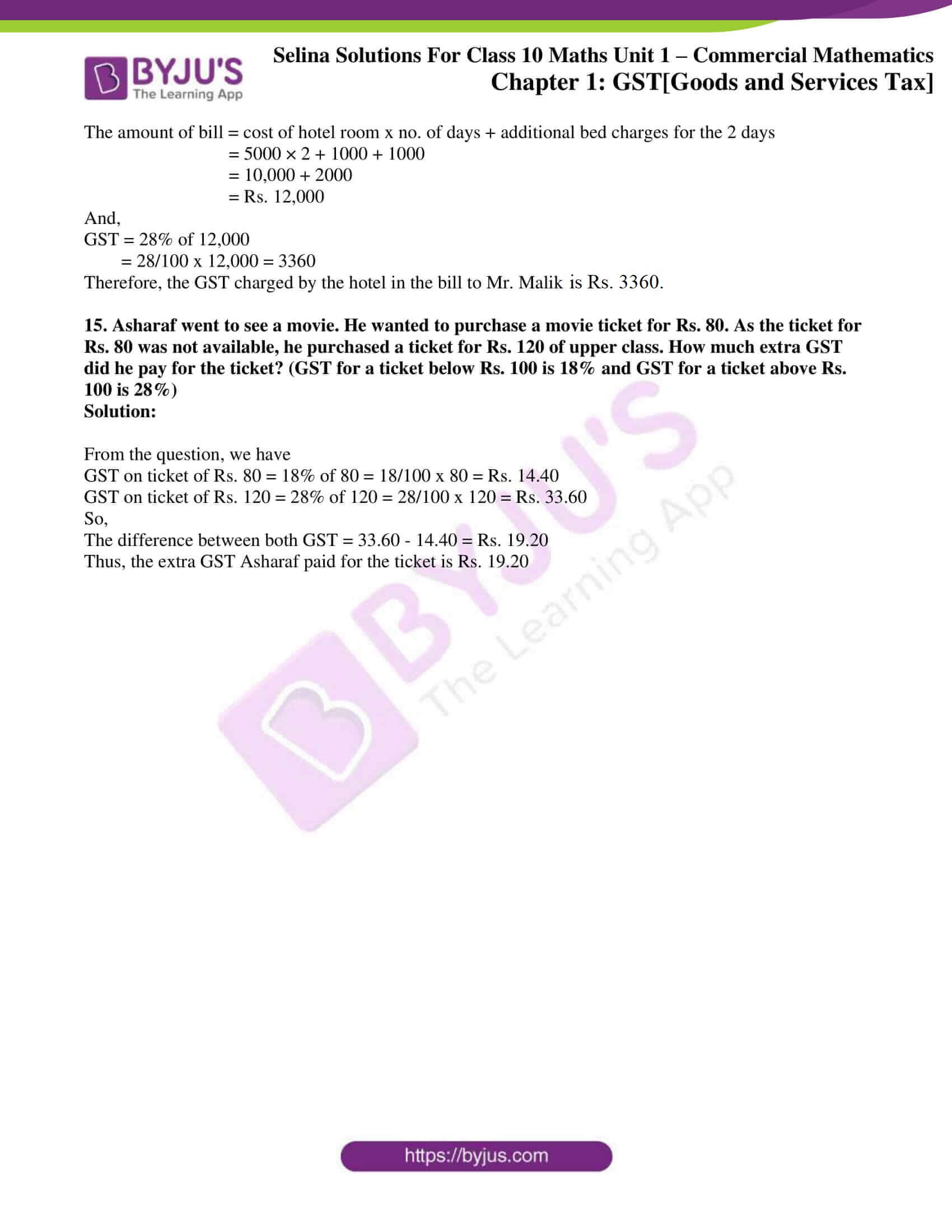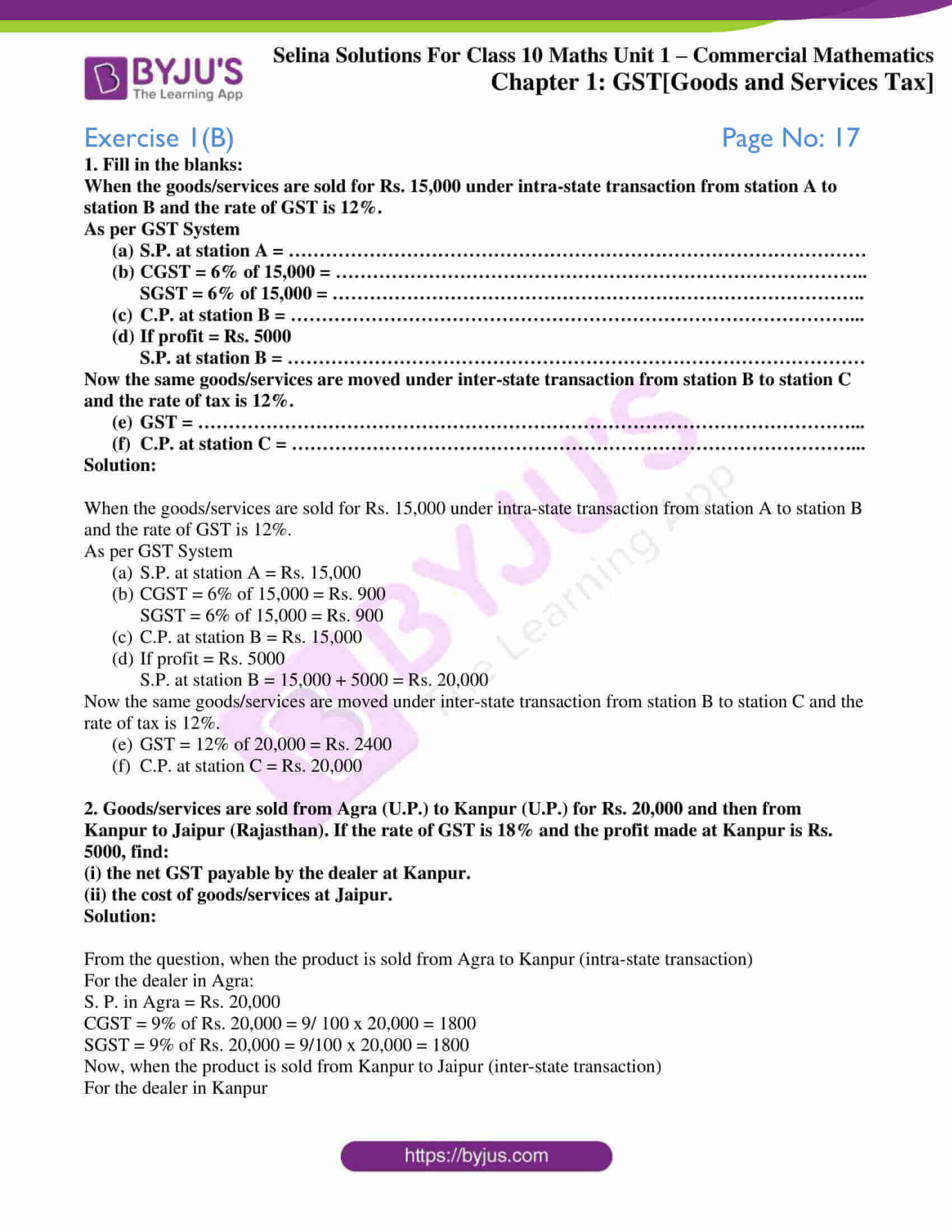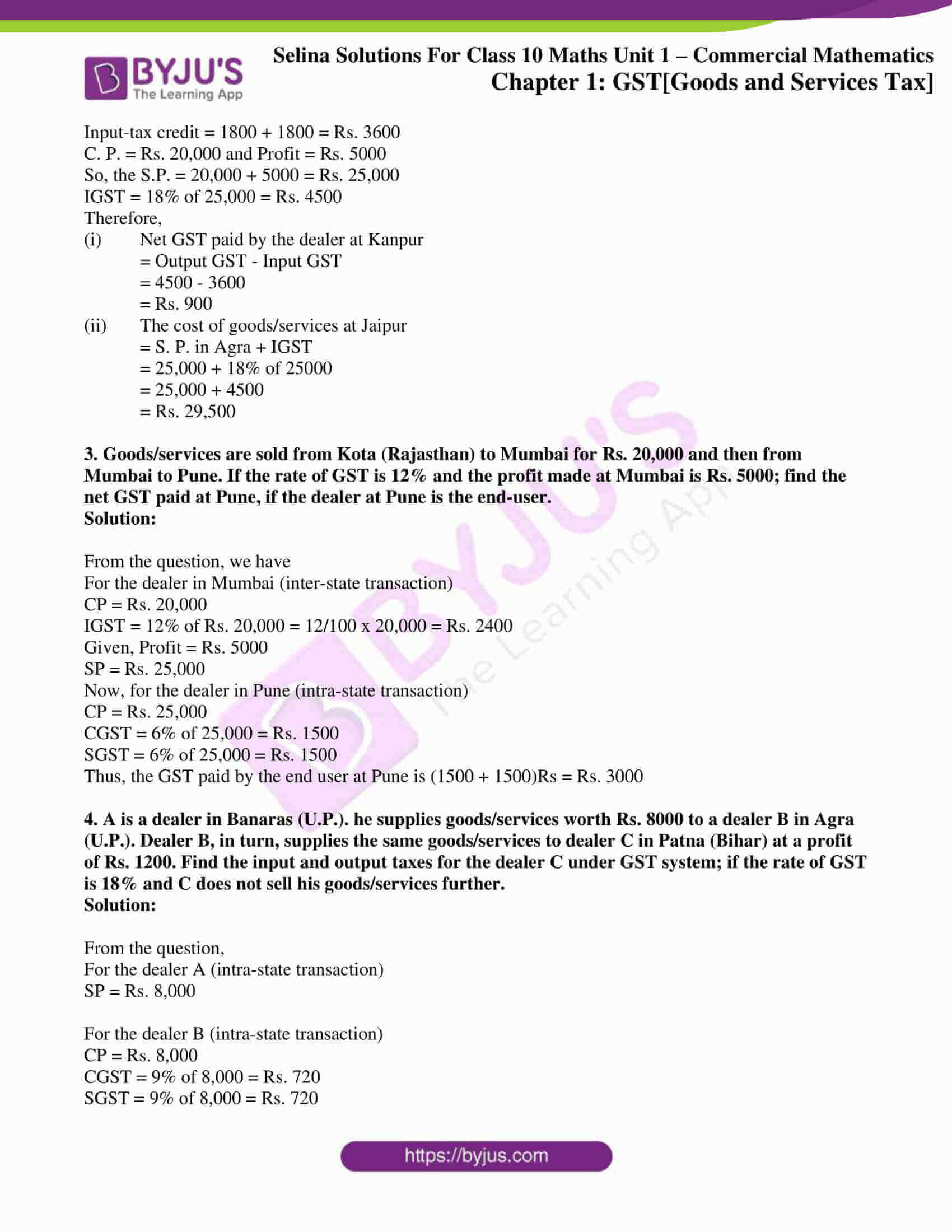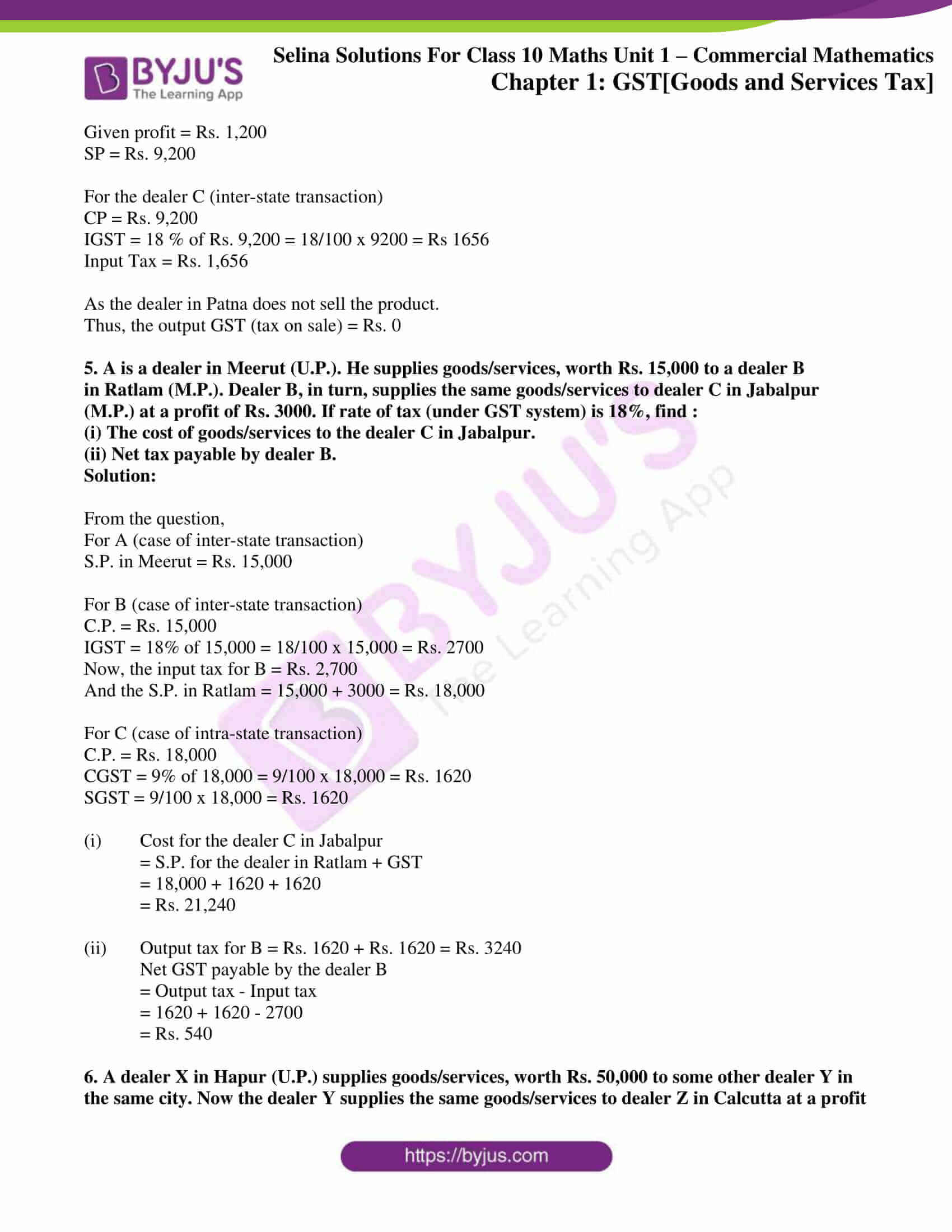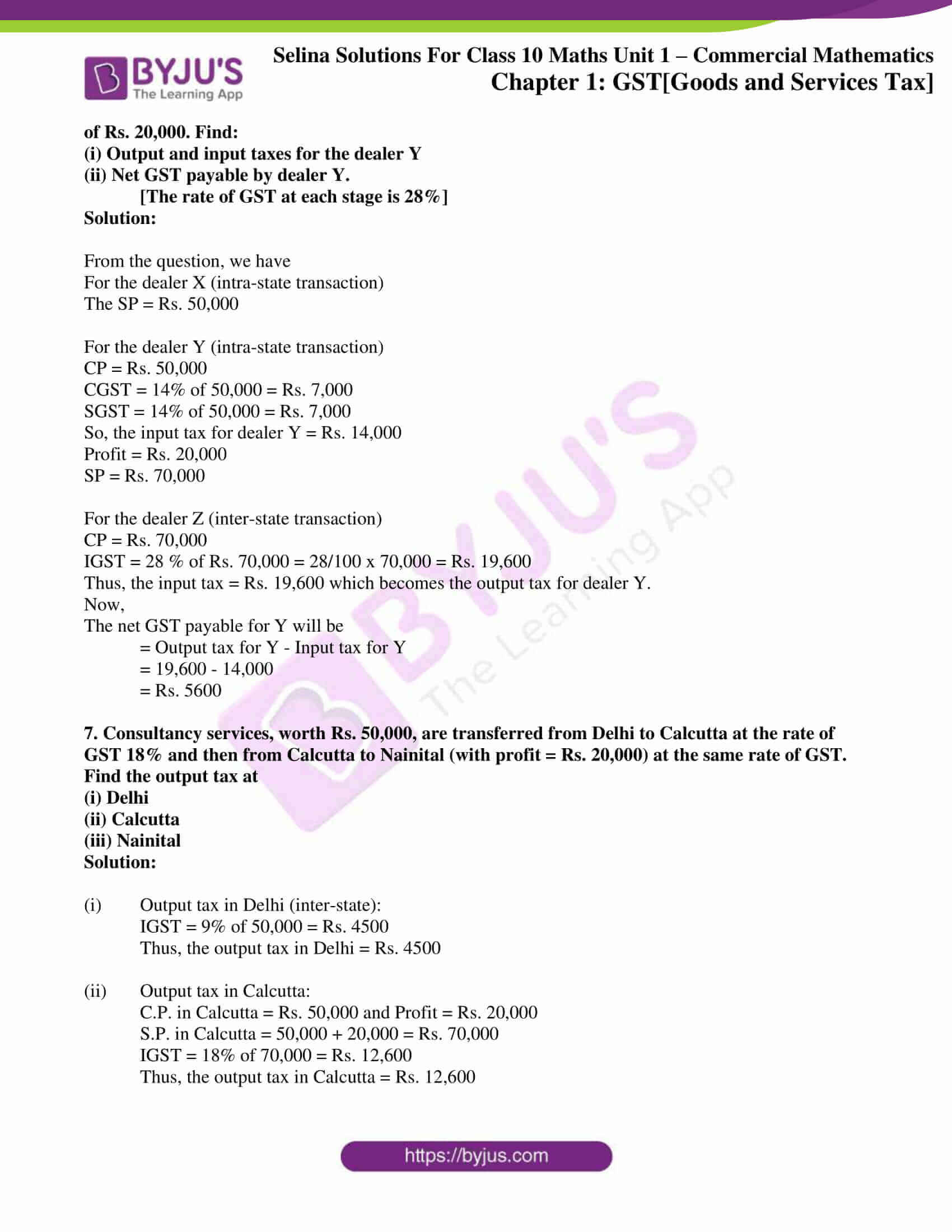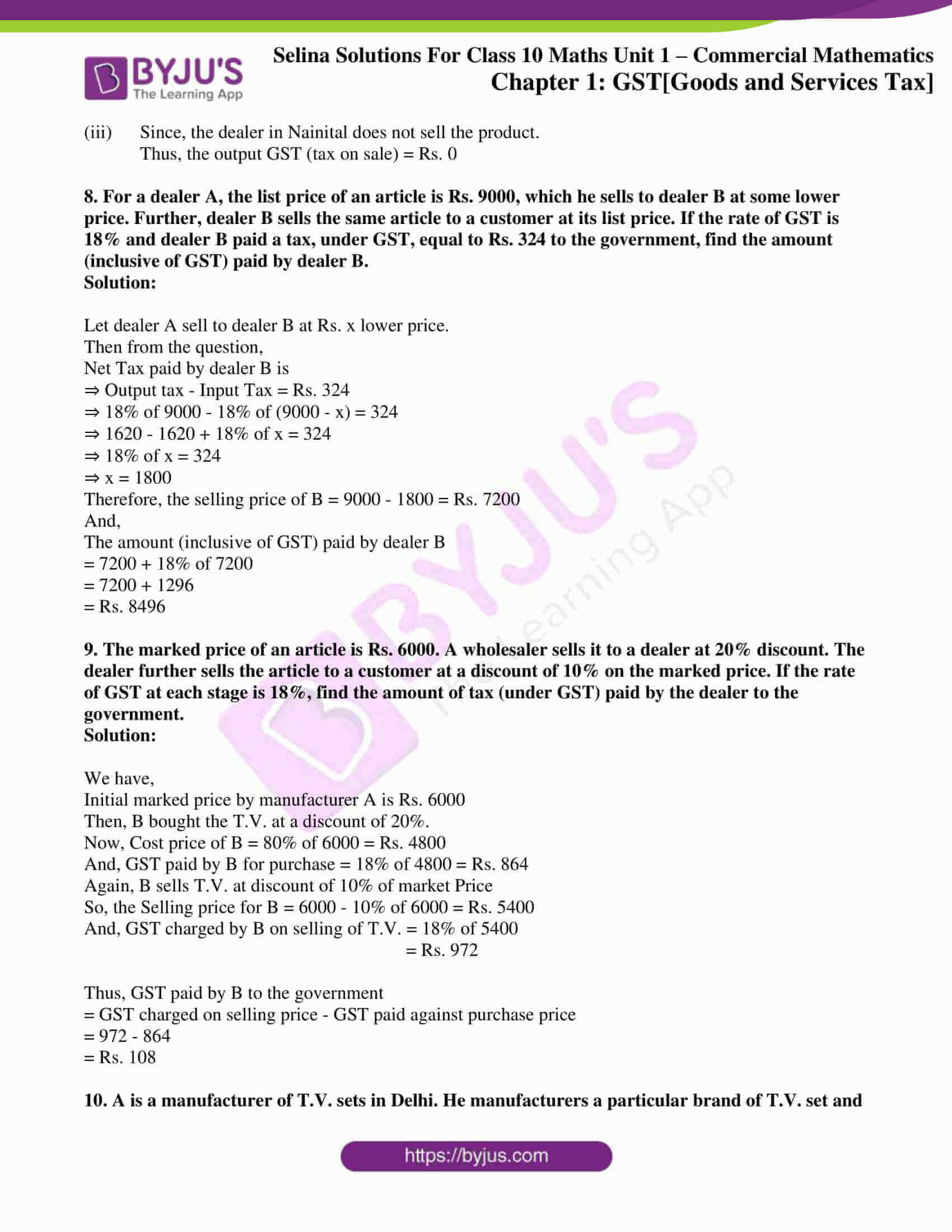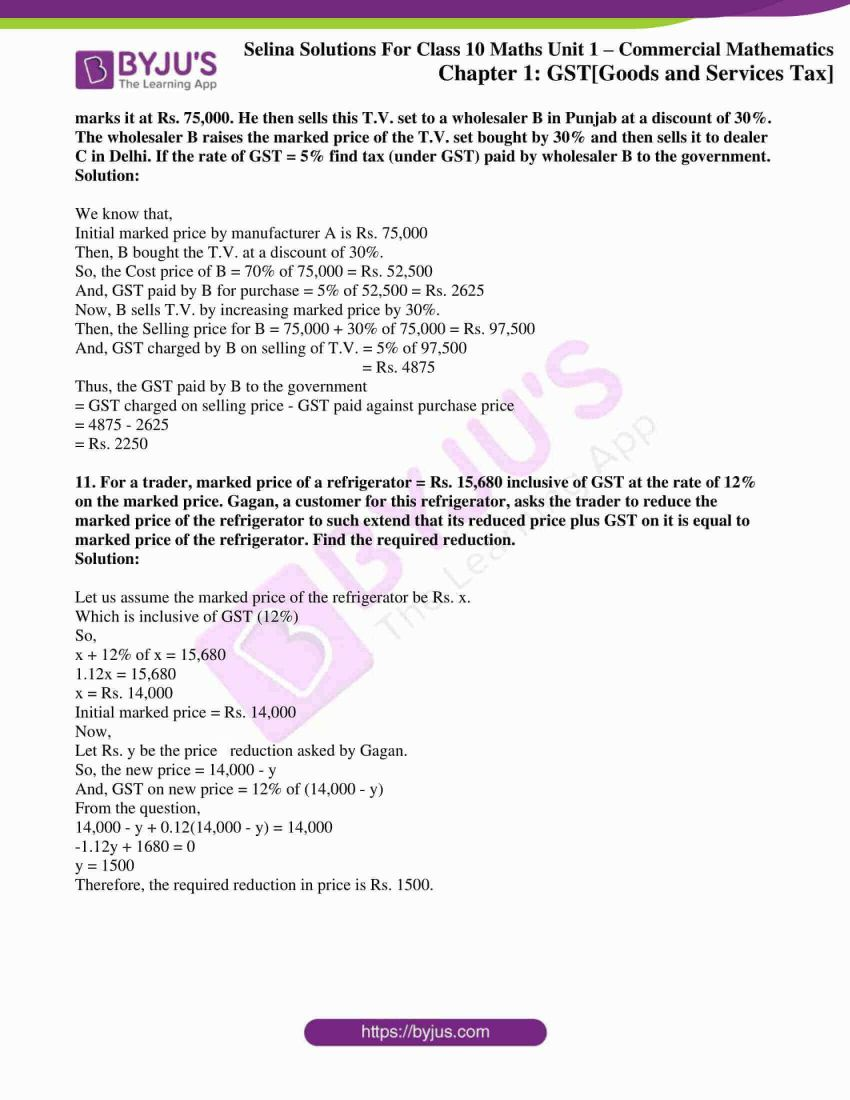## Access Selina Solutions Concise Maths Class 10 Chapter 1 GST [Goods and Services Tax]

### Exercise 1(A) Page No: 8

1. For the following transaction within Delhi, fill in the blanks to find the amount of bill:

MRP = Rs 12,000, Discount % = 30%, GST = 18%

Discount =

Selling price (discounted value) =

CGST =

SGST =

IGST =

Amount of Bill =

Solution:

Given,

MRP = Rs 12,000, Discount % = 30%, GST = 18%

Now,

Discount = 30% of 12,000 = (30/100) x 1200 = Rs 3600

So,

Selling price (discounted value) = 12000 – 3600 = Rs 8400

CGST = 9% of 8400 = Rs 756

SGST = 9% of 8400 = Rs 756

IGST = 0

Thus, the amount of bill = Selling price + CGST + SGST

= 8400 + 756 + 756

= Rs 9912

2. For the following transaction from Delhi to Jaipur, fill in the blanks to find the amount of bill:

MRP = Rs 50,000, Discount % = 20%, GST = 28%

Discount =

Selling price (discounted value) =

CGST =

SGST =

IGST =

Amount of Bill =

Solution:

Given,

MRP = Rs 50,000, Discount % = 20%, GST = 28%

Now,

Discount = 20% of 50,000 = (20/100) x 50,000 = Rs 10,000

So,

Selling price (discounted value) = 50,000 – 10,000 = Rs 40,000

CGST = 0

SGST = 0

IGST = 28% of 40,000 = (28/100) x 40,000 = Rs 11,200

Thus, the amount of bill = Selling price + IGST

= 40,000 + 11,200

= Rs 51,200

3. A computer mechanic in Delhi charges repairing cost from five different persons A, B, C, D and E with certain discounts. The repairing costs and the corresponding discounts are as given below:

 Name of the person A B C D E Repairing cost (in Rs) 5500 6250 4800 7200 3500 Discount % 30 40 30 20 40

If the rate of GST is 18%, find the total money (including GST) received by the mechanic.

Solution:

 Name of the person Repairing cost (in Rs) Discount % Discount Selling price CGST (9%) SGST (9%) A 5500 30 1650 3850 346.5 346.5 B 6250 40 2500 3750 337.5 337.5 C 4800 30 1440 3360 302.4 302.4 D 7200 20 1440 5760 518.4 518.4 E 3500 40 1400 2100 189 189 Total 18,820 1693.8 1693.8

Thus,

The total money (including GST) received by the mechanic is 18,820 + 1693.8 + 1693.8 = Rs. 22,207.60

4. Find the amount of bill for the following intra-state transaction of goods/services. The GST rate is 5%.

 Quantity (no. of items) MRP of each item (in Rs.) Discount % 18 150 10 24 240 20 30 100 30 12 120 20

Solution:

 Quantity MRP Total MRP Discount % Discounted price Selling price CGST 2.5% SGST 2.5% 18 150 2700 10 270 2430 60.75 60.75 24 240 5760 20 1152 4608 115.2 115.2 30 100 3000 30 900 2100 52.5 52.5 12 120 1440 20 288 1152 28.8 28.8 Total 10,290 257.25 257.25

Thus,

The amount of bill = Selling price + GST

= 10,290 + 257.25 + 257.25

= Rs. 10,804.5

5. Find the amount of bill for the following inter-state transaction of goods/services. The GST rate is 18%.

 Quantity (no. of items) 35 47 20 MRP of each item (in Rs.) 420 600 350 Discount % 10 10 20

Solution:

 Quantity MRP Total MRP Discount % Discounted price Selling price CGST 9% SGST 9% 35 420 14,700 10 1470 13,230 1190.7 1190.7 47 600 28,200 10 2820 25,380 2284.2 2284.2 20 350 7000 20 1400 5600 504 504 Total 44,210 3978.9 3978.9

Thus,

The amount of bill = Selling price + CGST + SGST

= 44,210 + 3978.9 + 3978.9

= Rs. 52,167.80

6. Find the amount of bill for the following intra-state transaction of goods/services.

 MRP (in Rs.) 12,000 15,000 9500 18,000 Discount % 30 20 30 40 CGST % 6 9 14 2.5

Solution:

 MRP (in Rs.) Discount % CGST % Discounted value Selling price CGST SGST 12,000 30 6 3600 8400 504 504 15,000 20 9 3000 12,000 1080 1080 9500 30 14 2850 6650 931 931 18,000 40 2.5 7200 10,800 270 270 37,850 2785 2785

Thus, the amount of bill = Selling price + CGST + SGST

= 37,850 + 2785 + 2785 = Rs. 43,420

7. For the data given above in questions no. 6, find the amount of bill for the inter-state transaction.

 MRP (in Rs.) 12,000 15,000 9500 18,000 Discount % 30 20 30 40 CGST % 6 9 14 2.5

Solution:

 MRP (in Rs.) Discount Discounted value Selling price IGST IGST 12,000 30 3600 8400 12 1008 15,000 20 3000 12,000 18 2160 9500 30 2850 6650 28 1862 18,000 40 7200 10,800 5 540 37,850 5570

Thus, the amount of bill = Selling price + GST

= 37,850 + 5570

= Rs. 43,420

8. A dealer in Mumbai supplied some items at the following prices to a dealer in Delhi. Find the total amount of the bill.

 Rate per piece (in Rs.) Quantity (no. of pieces) Discount % SGST % 180 10 – 9 260 20 20 9 310 30 – 9 175 20 30 9

Solution:

 Rate per piece (in Rs.) Quantity (no. of pieces) Discount % MRP Selling price IGST 18% 180 10 Net 1800 1800 324 260 20 20 5200 4160 748.8 310 30 Net 9300 9300 1674 175 20 30 3500 2450 441 17,710 3187.8

Thus, the amount of bill = Selling price + IGST

= 17,710 + 3187.8

= Rs. 20,897.80

9. National Trading Company, Meerut (UP) made the supply of the following goods/services to Samarth Traders, Noida (UP). Find the total amount of bill if the rate of GST = 12%

 Quantity (no. of pieces) 20 30 12 40 MRP (in Rs. per piece) 225 320 300 250 Discount % 40 30 50 40

Solution:

 MRP (in Rs. per piece) Quantity (no. of pieces) Discount % MRP Selling price SGST 6% CGST 6% 225 20 40 4500 2700 162 162 320 30 30 9600 6720 403.2 403.2 300 12 50 3600 1800 108 108 250 40 40 10,000 6000 360 360 17,220 1033.2 1033.2

Thus, the amount of bill = Selling price + SGST + CGST

= 17,220 + 2066.4

= Rs. 19,286.4

10. M/s Ram Traders, Delhi, provided the following services to M/s Geeta Trading Company in Agra (UP). Find the amount of bill:

 Number of services 8 12 10 16 Cost of each service (in Rs.) 680 320 260 420 GST % 5 12 18 12

Solution:

 Number of services Cost of each service (in Rs.) GST % MRP IGST 8 680 5 5440 272 12 320 12 3840 460.8 10 260 18 2600 468 16 420 12 6720 806.4 18,600 2007.2

Thus, the amount of bill = Selling price + IGST

= 18,600 + 2007.2

= Rs. 20,607.2

11. For the following, find the amount of bill data:

 Rate per piece (in Rs.) Number of pieces Discount % GST% 18 360 10 12 12 480 20 18 12 120 5 12 28 150 20 28

Solution:

 Rate per piece (in Rs.) Number of pieces Discount % MRP (in Rs.) Selling price (in Rs.) GST % GST (in Rs.) 18 360 10 6480 5832 12 699.84 12 480 20 5760 4608 18 829.44 12 120 5 1440 1368 12 164.16 28 150 20 4200 3360 28 940.8 15,168 2634.24

Thus, the amount of bill = Selling price + GST

= 15,168 + 2634.24

= Rs. 17,802.24

12. The tax invoice of a telecom service in Meerut shows cost of services provided by it as Rs. 750. If the GST rate is 18%, find the amount of the bill.

Solution:

From the question, we have

GST = 18% of 750

= 18/100 x 750 = Rs. 135

Thus, the amount of bill = 750 + 135 = Rs. 885

13. Mr. Pankaj took Health Insurance Policy for his family and paid Rs. 900 as SGST. Find the total annual premium paid by him for this policy, rate of GST being 18%.

Solution:

Let’s consider that the total annual premium paid by Mr. Pankaj be Rs. X.

Then, from the question

18% of X = SGST + CGST

18% of X = 1800  [as SGST = CGST]

So, 18/100 x X = 1800

X = Rs. 10,000

Therefore, the total annual premium paid by him for the policy is Rs. 10,000

14. Mr. Malik went on a tour to Goa. He took a room in a hotel for two days at the rate of Rs. 5000 per day. On the same day, his friend John also joined him. Hotel provided an extra bed charging Rs. 1000 per day for the bed. How much GST, at the rate of 28% is charged by the hotel in the bill to Mr. Malik for both the days?

Solution:

From the question, we have

The amount of bill = cost of hotel room x no. of days + additional bed charges for the 2 days

= 5000 × 2 + 1000 + 1000

= 10,000 + 2000

= Rs. 12,000

And,

GST = 28% of 12,000

= 28/100 x 12,000 = 3360

Therefore, the GST charged by the hotel in the bill to Mr. Malik is Rs. 3360.

15. Asharaf went to see a movie. He wanted to purchase a movie ticket for Rs. 80. As the ticket for Rs. 80 was not available, he purchased a ticket for Rs. 120 of upper class. How much extra GST did he pay for the ticket? (GST for a ticket below Rs. 100 is 18% and GST for a ticket above Rs. 100 is 28%)

Solution:

From the question, we have

GST on ticket of Rs. 80 = 18% of 80 = 18/100 x 80 = Rs. 14.40

GST on ticket of Rs. 120 = 28% of 120 = 28/100 x 120 = Rs. 33.60

So,

The difference between both GST = 33.60 – 14.40 = Rs. 19.20

Thus, the extra GST Asharaf paid for the ticket is Rs. 19.20

### Exercise 1(B) Page No: 17

1. Fill in the blanks:

When the goods/services are sold for Rs. 15,000 under intra-state transaction from station A to station B and the rate of GST is 12%.

As per GST System

(a) S.P. at station A = …………………………………………………………………………………

(b) CGST = 6% of 15,000 = …………………………………………………………………………..
SGST = 6% of 15,000 = …………………………………………………………………………..

(c) C.P. at station B = …………………………………………………………………………………

(d) If profit = Rs. 5000
S.P. at station B = …………………………………………………………………………………

Now the same goods/services are moved under inter-state transaction from station B to station C and the rate of tax is 12%.

(e) GST = ………………………………………………………………………………………………

(f) C.P. at station C = …………………………………………………………………………………

Solution:

When the goods/services are sold for Rs. 15,000 under intra-state transaction from station A to station B and the rate of GST is 12%.

As per GST System

(a) S.P. at station A = Rs. 15,000

(b) CGST = 6% of 15,000 = Rs. 900

SGST = 6% of 15,000 = Rs. 900

(c) C.P. at station B = Rs. 15,000

(d) If profit = Rs. 5000

S.P. at station B = 15,000 + 5000 = Rs. 20,000

Now the same goods/services are moved under inter-state transaction from station B to station C and the rate of tax is 12%.

(e) GST = 12% of 20,000 = Rs. 2400

(f) C.P. at station C = Rs. 20,000

2. Goods/services are sold from Agra (U.P.) to Kanpur (U.P.) for Rs. 20,000 and then from Kanpur to Jaipur (Rajasthan). If the rate of GST is 18% and the profit made at Kanpur is Rs. 5000, find:

(i) the net GST payable by the dealer at Kanpur.

(ii) the cost of goods/services at Jaipur.

Solution:

From the question, when the product is sold from Agra to Kanpur (intra-state transaction)

For the dealer in Agra:

S. P. in Agra = Rs. 20,000

CGST = 9% of Rs. 20,000 = 9/ 100 x 20,000 = 1800

SGST = 9% of Rs. 20,000 = 9/100 x 20,000 = 1800

Now, when the product is sold from Kanpur to Jaipur (inter-state transaction)

For the dealer in Kanpur

Input-tax credit = 1800 + 1800 = Rs. 3600

C. P. = Rs. 20,000 and Profit = Rs. 5000

So, the S.P. = 20,000 + 5000 = Rs. 25,000

IGST = 18% of 25,000 = Rs. 4500

Therefore,

(i) Net GST paid by the dealer at Kanpur

= Output GST – Input GST

= 4500 – 3600

= Rs. 900

(ii) The cost of goods/services at Jaipur

= S. P. in Agra + IGST

= 25,000 + 18% of 25000

= 25,000 + 4500

= Rs. 29,500

3. Goods/services are sold from Kota (Rajasthan) to Mumbai for Rs. 20,000 and then from Mumbai to Pune. If the rate of GST is 12% and the profit made at Mumbai is Rs. 5000; find the net GST paid at Pune, if the dealer at Pune is the end-user.

Solution:

From the question, we have

For the dealer in Mumbai (inter-state transaction)

CP = Rs. 20,000

IGST = 12% of Rs. 20,000 = 12/100 x 20,000 = Rs. 2400

Given, Profit = Rs. 5000

SP = Rs. 25,000

Now, for the dealer in Pune (intra-state transaction)

CP = Rs. 25,000

CGST = 6% of 25,000 = Rs. 1500

SGST = 6% of 25,000 = Rs. 1500

Thus, the GST paid by the end user at Pune is (1500 + 1500)Rs = Rs. 3000

4. A is a dealer in Banaras (U.P.). he supplies goods/services worth Rs. 8000 to a dealer B in Agra (U.P.). Dealer B, in turn, supplies the same goods/services to dealer C in Patna (Bihar) at a profit of Rs. 1200. Find the input and output taxes for the dealer C under GST system; if the rate of GST is 18% and C does not sell his goods/services further.

Solution:

From the question,

For the dealer A (intra-state transaction)

SP = Rs. 8,000

For the dealer B (intra-state transaction)

CP = Rs. 8,000

CGST = 9% of 8,000 = Rs. 720

SGST = 9% of 8,000 = Rs. 720

Given profit = Rs. 1,200

SP = Rs. 9,200

For the dealer C (inter-state transaction)

CP = Rs. 9,200

IGST = 18 % of Rs. 9,200 = 18/100 x 9200 = Rs 1656

Input Tax = Rs. 1,656

As the dealer in Patna does not sell the product.

Thus, the output GST (tax on sale) = Rs. 0

5. A is a dealer in Meerut (U.P.). He supplies goods/services, worth Rs. 15,000 to a dealer B in Ratlam (M.P.). Dealer B, in turn, supplies the same goods/services to dealer C in Jabalpur (M.P.) at a profit of Rs. 3000. If rate of tax (under GST system) is 18%, find :

(i) The cost of goods/services to the dealer C in Jabalpur.

(ii) Net tax payable by dealer B.

Solution:

From the question,

For A (case of inter-state transaction)

S.P. in Meerut = Rs. 15,000

For B (case of inter-state transaction)

C.P. = Rs. 15,000

IGST = 18% of 15,000 = 18/100 x 15,000 = Rs. 2700

Now, the input tax for B = Rs. 2,700

And the S.P. in Ratlam = 15,000 + 3000 = Rs. 18,000

For C (case of intra-state transaction)

C.P. = Rs. 18,000

CGST = 9% of 18,000 = 9/100 x 18,000 = Rs. 1620

SGST = 9/100 x 18,000 = Rs. 1620

(i) Cost for the dealer C in Jabalpur

= S.P. for the dealer in Ratlam + GST

= 18,000 + 1620 + 1620

= Rs. 21,240

(ii) Output tax for B = Rs. 1620 + Rs. 1620 = Rs. 3240

Net GST payable by the dealer B

= Output tax – Input tax

= 1620 + 1620 – 2700

= Rs. 540

6. A dealer X in Hapur (U.P.) supplies goods/services, worth Rs. 50,000 to some other dealer Y in the same city. Now the dealer Y supplies the same goods/services to dealer Z in Calcutta at a profit of Rs. 20,000. Find:

(i) Output and input taxes for the dealer Y

(ii) Net GST payable by dealer Y.

[The rate of GST at each stage is 28%]

Solution:

From the question, we have

For the dealer X (intra-state transaction)

The SP = Rs. 50,000

For the dealer Y (intra-state transaction)

CP = Rs. 50,000

CGST = 14% of 50,000 = Rs. 7,000

SGST = 14% of 50,000 = Rs. 7,000

So, the input tax for dealer Y = Rs. 14,000

Profit = Rs. 20,000

SP = Rs. 70,000

For the dealer Z (inter-state transaction)

CP = Rs. 70,000

IGST = 28 % of Rs. 70,000 = 28/100 x 70,000 = Rs. 19,600

Thus, the input tax = Rs. 19,600 which becomes the output tax for dealer Y.

Now,

The net GST payable for Y will be

= Output tax for Y – Input tax for Y

= 19,600 – 14,000

= Rs. 5600

7. Consultancy services, worth Rs. 50,000, are transferred from Delhi to Calcutta at the rate of GST 18% and then from Calcutta to Nainital (with profit = Rs. 20,000) at the same rate of GST. Find the output tax at

(i) Delhi

(ii) Calcutta

(iii) Nainital

Solution:

(i) Output tax in Delhi (inter-state):

IGST = 9% of 50,000 = Rs. 4500

Thus, the output tax in Delhi = Rs. 4500

(ii) Output tax in Calcutta:

C.P. in Calcutta = Rs. 50,000 and Profit = Rs. 20,000

S.P. in Calcutta = 50,000 + 20,000 = Rs. 70,000

IGST = 18% of 70,000 = Rs. 12,600

Thus, the output tax in Calcutta = Rs. 12,600

(iii) Since, the dealer in Nainital does not sell the product.

Thus, the output GST (tax on sale) = Rs. 0

8. For a dealer A, the list price of an article is Rs. 9000, which he sells to dealer B at some lower price. Further, dealer B sells the same article to a customer at its list price. If the rate of GST is 18% and dealer B paid a tax, under GST, equal to Rs. 324 to the government, find the amount (inclusive of GST) paid by dealer B.

Solution:

Let dealer A sell to dealer B at Rs. x lower price.

Then from the question,

Net Tax paid by dealer B is

⇒ Output tax – Input Tax = Rs. 324

⇒ 18% of 9000 – 18% of (9000 – x) = 324

⇒ 1620 – 1620 + 18% of x = 324

⇒ 18% of x = 324

⇒ x = 1800

Therefore, the selling price of B = 9000 – 1800 = Rs. 7200

And,

The amount (inclusive of GST) paid by dealer B

= 7200 + 18% of 7200

= 7200 + 1296

= Rs. 8496

9. The marked price of an article is Rs. 6000. A wholesaler sells it to a dealer at 20% discount. The dealer further sells the article to a customer at a discount of 10% on the marked price. If the rate of GST at each stage is 18%, find the amount of tax (under GST) paid by the dealer to the government.

Solution:

We have,

Initial marked price by manufacturer A is Rs. 6000

Then, B bought the T.V. at a discount of 20%.

Now, Cost price of B = 80% of 6000 = Rs. 4800

And, GST paid by B for purchase = 18% of 4800 = Rs. 864

Again, B sells T.V. at discount of 10% of market Price

So, the Selling price for B = 6000 – 10% of 6000 = Rs. 5400

And, GST charged by B on selling of T.V. = 18% of 5400

= Rs. 972

Thus, GST paid by B to the government

= GST charged on selling price – GST paid against purchase price

= 972 – 864

= Rs. 108

10. A is a manufacturer of T.V. sets in Delhi. He manufacturers a particular brand of T.V. set and marks it at Rs. 75,000. He then sells this T.V. set to a wholesaler B in Punjab at a discount of 30%. The wholesaler B raises the marked price of the T.V. set bought by 30% and then sells it to dealer C in Delhi. If the rate of GST = 5% find tax (under GST) paid by wholesaler B to the government.

Solution:

We know that,

Initial marked price by manufacturer A is Rs. 75,000

Then, B bought the T.V. at a discount of 30%.

So, the Cost price of B = 70% of 75,000 = Rs. 52,500

And, GST paid by B for purchase = 5% of 52,500 = Rs. 2625

Now, B sells T.V. by increasing marked price by 30%.

Then, the Selling price for B = 75,000 + 30% of 75,000 = Rs. 97,500

And, GST charged by B on selling of T.V. = 5% of 97,500

= Rs. 4875

Thus, the GST paid by B to the government

= GST charged on selling price – GST paid against purchase price

= 4875 – 2625

= Rs. 2250

11. For a trader, marked price of a refrigerator = Rs. 15,680 inclusive of GST at the rate of 12% on the marked price. Gagan, a customer for this refrigerator, asks the trader to reduce the marked price of the refrigerator to such extend that its reduced price plus GST on it is equal to marked price of the refrigerator. Find the required reduction.

Solution:

Let us assume the marked price of the refrigerator be Rs. x.

Which is inclusive of GST (12%)

So,

x + 12% of x = 15,680

1.12x = 15,680

x = Rs. 14,000

Initial marked price = Rs. 14,000

Now,

Let Rs. y be the price reduction asked by Gagan.

So, the new price = 14,000 – y

And, GST on new price = 12% of (14,000 – y)

From the question,

14,000 – y + 0.12(14,000 – y) = 14,000

-1.12y + 1680 = 0

y = 1500

Therefore, the required reduction in price is Rs. 1500.